2.2. Origin of the Variations

At a fundamental level, the physical origin of variations is not known, although accretion-disk instabilities are probably involved. For example, Kawaguchi et al. 37 show that continuum variations with a PDS of the form of Eq. (7) can be explained by magnetohydrodynamic instabilities, specifically disconnection events, within the disk. Variable accretion rates have also been considered. In some specific atypical cases, variations have been attributed to variable obscuration of the nuclear source and to microlensing due to stars in the host galaxy. There are a number of important physical time scales that might be associated with variability. We include them here in the convenient form given by Edelson & Nandra 21. The first of these is the crossing time already mentioned in Eq. (6), which we rewrite here as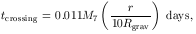(8)

which is the time it takes a radiative signal to cross the X-ray emitting region (assumed to be at r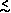10 Rgrav). Here M7 is the black-hole mass in units of 107 M. Variations might also be expected on the time scale of the orbital period,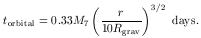(9)

Thermal instabilities might also cause variations on the time scale for their development,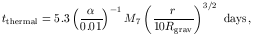(10)

whereis the viscosity parameter. Mechanical instabilities may propagate as acoustic waves, which will travel at the sound speed and thus cross the disk on a time scale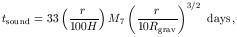(11)

where H is the disk thickness. And finally, the time scale over which the effects of variations in the accretion rate will propagate through the disk is given by the drift speed,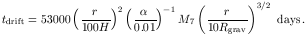(12)